Bug fix : DEplot bugs

There are a number of bugs and weaknesses affecting DEplot in the DEtools package.

> with(DEtools):

• It uses thick curves (thickness 3) to plot trajectories, even when thickness is specified as 0, 1 or 2.

> DEplot({diff(x(t),t)=1,diff(y(t),t)=x(t)},[x(t),y(t)],t=0..2,{[x(0)=0,y(0)=1]},linecolour=blue,thickness=1,
arrows=none);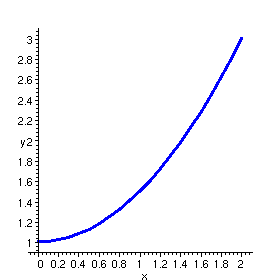A work-around is to use subs on the result of DEplot as follows:

> subs(THICKNESS(3)=THICKNESS(1),");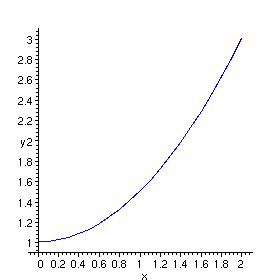This bug has been corrected in Release 5.

• It produces an error message in plots of 2 by 2 autonomous systems where the scene option involves the independent variable, and arrows=none is not specified.

> DEplot({diff(x(t),t)=1, diff(y(t),t)=x(t)}, [x(t),y(t)], t=0..1, [[x(0)=1,y(0)=1]], scene=[t,x]);

Error, (in DEtools/DEplot/CheckDE) invalid subscript selector

A direction field only makes sense here with scene=[x,y] . Nevertheless, you must specify arrows=none to indicate that you don't want it.

> DEplot({diff(x(t),t)=1, diff(y(t),t)=x(t)}, [x(t),y(t)], t=0..1, [[x(0)=1,y(0)=1]], scene=[t,x],arrows=none);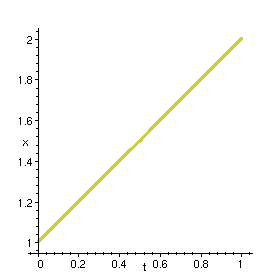This bug has been corrected in Release 5.

• The coords option, which allows the use of non-Cartesian coordinate systems in many other plotting commands, does not work here. In two-dimensional plots it causes an error message. In three-dimensional plots (with DEplot3d ) there is no error message, but the plot is done in Cartesian coordinates.

> DEplot({diff(r(t),t)=-sin(theta(t)/2), diff(theta(t),t)=1/2},
[r(t),theta(t)], t=0..3,
[[r(0)=1,theta(0)=0]], arrows=none,coords=polar);

Error, (in plot/options2d) unknown or bad argument, coords = polar

> DEplot3d({diff(r(t),t)=-sin(theta(t))/2, diff(theta(t),t)=1/2},
[r(t),theta(t)], t=-0..3,
[[r(0)=1,theta(0)=0]], scene=[r,theta,t],
coords=cylindrical);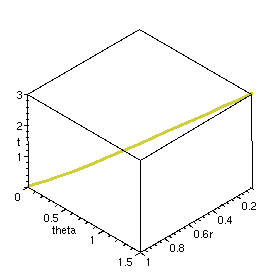A work-around is to produce a list of points, and plot it using plot (in two dimensions) or spacecurve (in three dimensions).

> L1:= op(indets(DEplot({diff(r(t),t)=-sin(theta(t)/2), diff(theta(t),t)=1/2},
[r(t),theta(t)], t=0 .. 3,
[[r(0)=1,theta(0)=0]],arrows=none),list(list(numeric)))):

> plot(L1,coords=polar,scaling=constrained);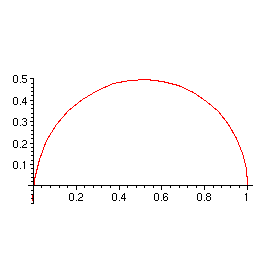> L2:= op(indets(DEplot3d({diff(r(t),t)=-sin(theta(t))/2, diff(theta(t),t)=1/2},
[r(t),theta(t)], t=-Pi..Pi,
[[r(0)=1,theta(0)=0]], scene=[r,theta,t]),list(list(numeric)))):

> plots[spacecurve](L2,coords=cylindrical);This can be done for the direction field too (if you don't mind a bit of distortion in the arrows). In order to have the direction field and trajectories in different colours, they should be plotted separately and combined using display .

> L3:= {op(indets(DEplot({diff(r(t),t)=-sin(theta(t)/2), diff(theta(t),t)=1/2},
[r(t),theta(t)], t=0 .. 3, r=0.1 .. 1.1,theta=0 .. 1.55, dirgrid=[10,8]),list(list(numeric))))}:

> p1:= plot(L1, coords=polar, colour=blue):
p2:= plot(L3, coords=polar, colour=black):
plots[display]({p1,p2},scaling=constrained,axes=box);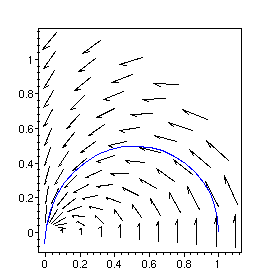• When the right side of one of the differential equations is identically 0, the direction field can not be plotted. With no initial conditions, there is an error message. With initial conditions, solution curves are plotted correctly but there are no arrows.

> DEplot({diff(x(t),t) = y(t)*x(t), diff(y(t),t) = 0}, [x(t),y(t)], t=0..0.5, x=0..1, y=-1..1, arrows=SMALL);

Error, (in DEplot) Cannot produce plot, non-autonomous DE(s) require initial conditions.

> DEplot({diff(x(t),t) = y(t), diff(y(t),t) = 0}, [x(t),y(t)], t=0 .. 0.5, {[x(0)=.5,y(0)=.5]}, x=0..1, y=-1..1, arrows=SMALL);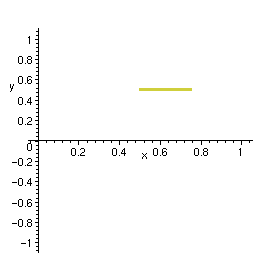A work-around is to use a very small but nonzero value for the derivative.

> DEplot({diff(x(t),t) = y(t), diff(y(t),t) = 0.0001*y(t)}, [x(t),y(t)], t=0 .. 0.5, {[x(0)=.5,y(0)=.5]}, x=0..1, y=-1..1, arrows=SMALL);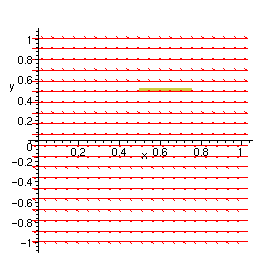• The direction field plotter does not work for differential equations that are not of the form derivative = expression.

> DEplot(diff(y(t),t) + y(t) = 1, y(t), t=0..1, [[y(0)=2]]);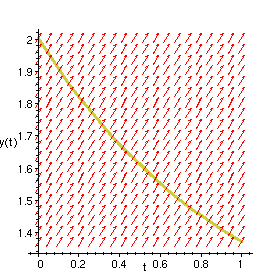The work-around is to rewrite the differential equations to put everything except derivatives on the right side.

> DEplot(diff(y(t),t) = 1 - y(t), y(t), t=0..1, [[y(0)=2]]);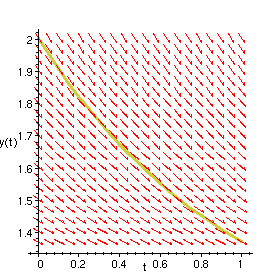This bug has been corrected in Release 5.

• In some circumstances, the direction field plotter fails to do an evalf , with the result that some of the arrows to be plotted contain symbolic expressions.

> DEplot(diff(y(t),t) = exp(1-2*t), y(t), t = 0 .. 1,y=0..1);

Plotting error, non-numeric vertex definition

In this case, the problem doesn't occur if a floating-point value is placed inside the exp .

> DEplot(diff(y(t),t) = exp(1.0-2*t), y(t), t = 0 .. 1,y=0..1);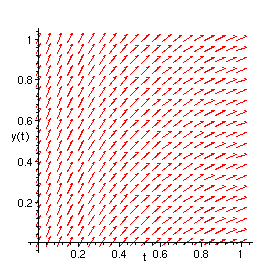Another work-around is to map evalf into every CURVES structure in the DEplot result.

> pl:= DEplot(diff(y(t),t) = exp(1-2*t), y(t), t = 0 .. 1,y=0..1):
pts:= indets(pl,specfunc(anything,CURVES)):
s:= map(t -> (t = map(evalf,t)), pts):
subs(s,pl);